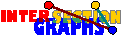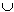# Intersection Bigraphs/Digraphs

In intersection bigraphs, the sets come in two flavors, say red and blue, and each set only recognizes intersection with sets of the different color. Therefore, the resulting intersection bigraph is bipartite. More formally, the intersection bigraph of a pair ((A,Sx/xU), (A,Ty/ yW)) of hypergraphs has V=UW as vertex set, and an edge between xU and yW whenever SxTy is nonempty.

See an example.

Intersection bigraphs contain less information than the corresponding intersection graphs. As for intersection multigraphs, the question whether this makes them easier or more difficult to recognize cannot be answered easily. It depends on the model. In some cases, the greater freedom enables us to represent every bigraph. For instance, it is easy to see that every bipartite graph is the intersection bigraph of subtrees of a tree, or convex sets in the plane.

There is only the following weak connection between intersection graphs and bigraphs: Let the bigraph B(G) of a graph G=(V,E) have V{xÇ/xV} as vertex set, and draw an edge between xV and yÇ, yV, whenever xyE or x=y.If G is intersection graph of P-sets, then B(G) is intersection bigraph of sets that are union of two intersecting P-sets.

Note that B(Ot) is the so-called `cocktail-party graph' CP(t), obtained from Kt,t by deleting a complete matching, which plays about the same role for bigraphs than the octahedrons for graphs.

### Intersection digraphs

The intersection digraph of a family (Sx,Tx), xV of pairs of sets has V as vertex set, and an arc from x to y whenever SxTy is nonempty.

This concept is older and more well-known than the concept of intersection bigraphs, however, intersection bigraphs are more general. The bigraph B(D) of a digraph D=(V,A) has V{xÇ/ xV} as vertex set. There is an edge between xV and yÇ, yV whenever xyA.[M97]: D is intersection digraph of pairs of P-sets if and only if B(D) is intersection bigraph of P-sets.

### Intersection bigraphs

For every intersection bigraph, we get again star graphs consisting of all vertices whose representative set covers some fixed single point. Surely the star graphs are complete bipartite.

What about the variants of our two evergreens? You may go to interval bigraphs or to line bigraphs.

Back to the start page for intersection graphs.

Erich Prisner